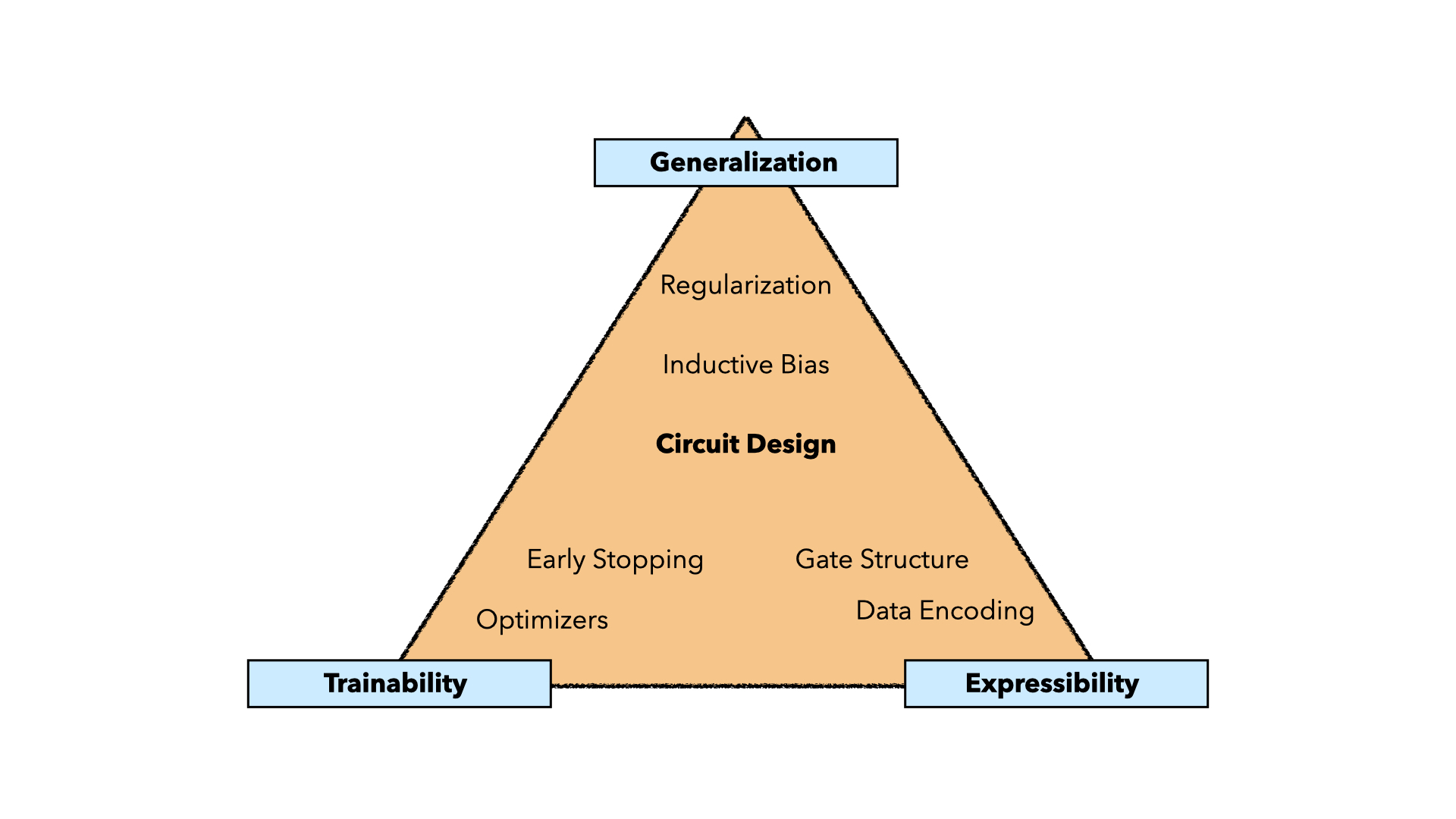Near-term quantum algorithms leverage the power of both quantum and classical resources in order to solve practical problems on today’s devices. The most prominent algorithm is called a VQA - Variational Quantum Algorithm - where the quantum component is a parametrized quantum circuit that outputs data that can be used to minimize a classical cost function. Below is a diagram of the VQA framework, which includes classical pre- and post-processing in order to design algorithms that can be run with short circuit depths and modest numbers of qubits. These algorithms can also be tailored to trapped-ion quantum computers, making them hardware-efficient.### Research directions

• Investigating quantum circuits: expressibility, generalization, trainability
• Designing parametrized quantum circuits as enhanced machine learning models
• Developing hardware-efficient techniques for achieving quantum advantage
• Producing new algorithms for practical applications

### Quantum circuits as ML models

Quantum circuits output probability distributions, making them a good candidate for unsupervised generative machine learning models. Unsupervised generative models take unlabelled training data and learn underlying patterns (according to some probability distribution) within the dataset. When sampled, the model operates as a generator, producing both samples from the training set as well as new similar samples that were not seen by the model. The latter behavior is known as generalization. While classically characterized, very few works focus on benchmarking this generalization within quantum circuits.

In our work, we focus on investigating quantum circuits for practical near-term applications - where one of the most prominent candidates is unsupervised generative machine learning models. We probe the model’s generalization capabilities, as well as the model’s expressibility (ability to express data) and the model’s trainability (ability to efficiently train with a classical computer). We utilize these insights in order to develop new algorithms that can be run on today’s devices, specifically tailored to trapped-ion quantum computers.